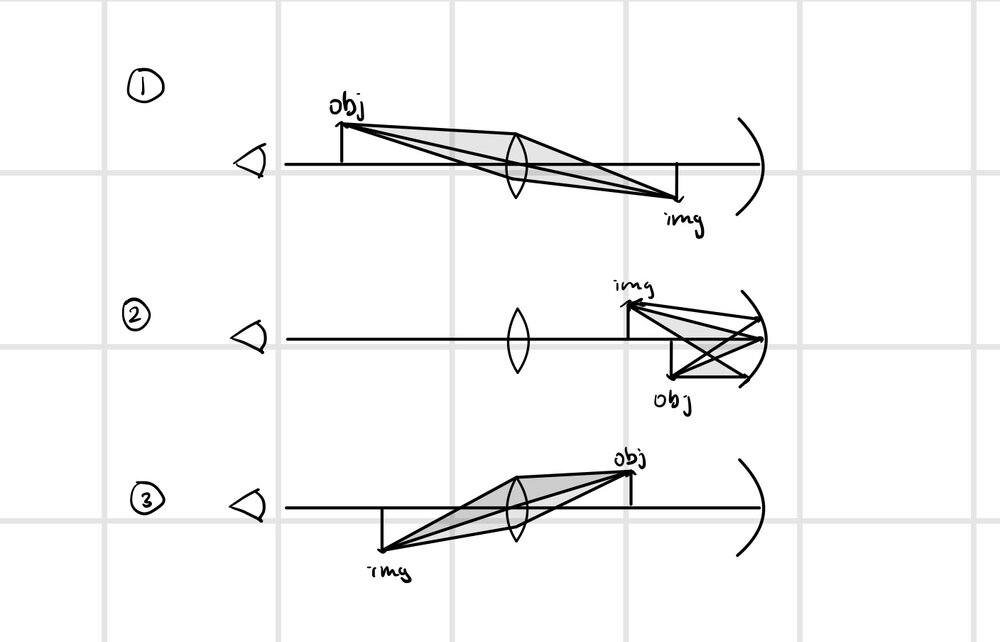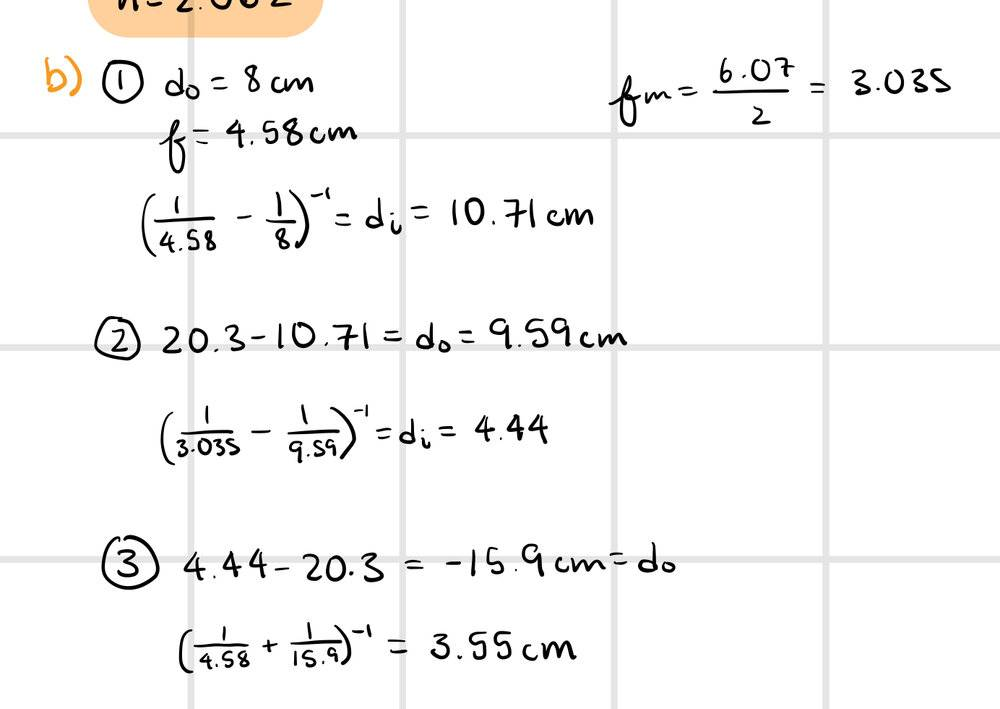# Combination of thin lens and concave mirror

physstudent189
Homework Statement:
The figure below shows a thin converging lens for which the radii are R1 = 8.48 cm and R2 = -11.4 cm. The lens is in front of a concave spherical mirror of radius R = 6.07 cm. If its focal points F1 and F2 are 4.58 cm from the vertex of the lens: b) If the lens and mirror are 20.3 cm apart and an object is placed 8.00 cm to the left of the lens, determine the position of the final image relative to the lens (Positive values are to the left).
Relevant Equations:
1/f = 1/do + 1/di
I created the following ray diagram to help me solve the problem:Then I applied the mirror equation 3 separate times.However, the final image distance I got is wrong. I'm wondering if I'm mistaken in taking the last object distance to be negative. However I only have one more try to get this right so I really want to make sure I'm approaching it from the right angle. I thought the last image distance would be negative because it is distance behind the converging lens, and we are told in the question that distances to the left of the lens (in front) are positive. I really appreciate any help or pointers anyone can give. Thank you!

physstudent189
nevermind I got it! Had to take the last obj. dist. as positive :-)

Homework Helper
Gold Member
2022 Award
Homework Statement:: The figure below shows a thin converging lens for which the radii are R1 = 8.48 cm and R2 = -11.4 cm. The lens is in front of a concave spherical mirror of radius R = 6.07 cm. If its focal points F1 and F2 are 4.58 cm from the vertex of the lens: b) If the lens and mirror are 20.3 cm apart and an object is placed 8.00 cm to the left of the lens, determine the position of the final image relative to the lens (Positive values are to the left).
Hi. There are a number of problems with the question.

The refractive index of the lens is missing. This makes it impossible to find the focal length of the lens.

A concave spherical mirror has only 1 focal point, so saying
“If its focal points F1 and F2 are 4.58 cm from the vertex of the lens”
makes no sense.

The question is incomplete. It looks like part a) has been omitted. It is often useful or necessary to see the whole question.

In your answer to b) ① you appear to be using the wrong value (4.58cm) for the focal length of the lens.

Edit. I'd already answered before I saw your post #2 saying you have solved the problem. But I would be very interested to know the resolution of the problems listed above!

•hutchphd